# 3-4-5 Triangle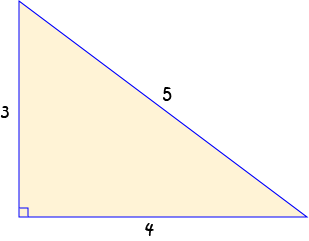A 3-4-5 triangle is a triangle with sides of length 3 units, 4 units and 5 units.

Any triangle with side lengths of 3 units, 4 units and 5 units is guaranteed to be a right-angled triangle.

## Constructing a 3-4-5 Triangle

This is a ruler and compass construction. We have to cheat a bit by measuring a few things, so it isn't quite a straight edge and compass construction.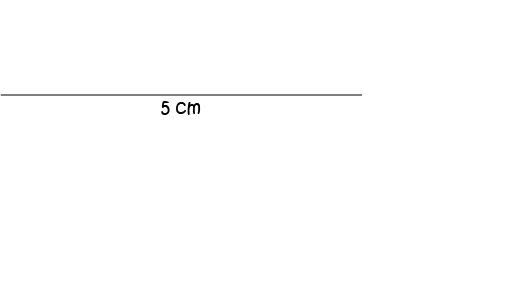Start off by drawing a line segment that's $5$ cm long. Don't be like Sam (you should see the mess he makes)! Use a ruler.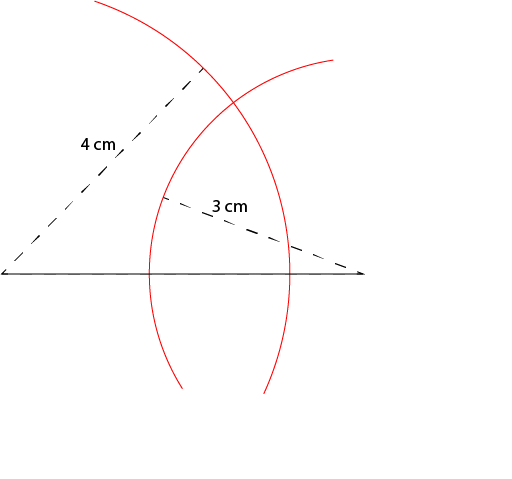Open out your compass to a radius of 4 centimetres. Stick the tip in at one end of your line segment and draw an arc. Make it nice and long so that it will intersect with the arc that you draw next.

Next, open your compass to a radius of 3 centimetres. Stick the tip in at the other end of your line segment and draw a second arc. Make it long enough to intersect with the first arc you drew.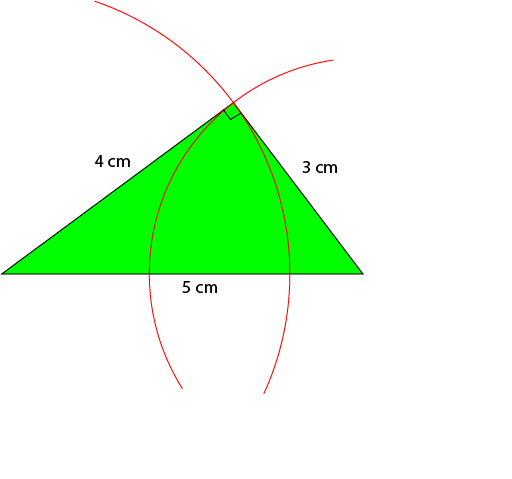Now you're ready to draw the sides of your triangle. Use your ruler to join up each end of the line segment to the point where the arcs intersect. The right angle will be between the two sides that you just formed. You have created your own, beautiful (if you used a ruler!), 3-4-5 triangle.

## Are There Any Other Side Lengths That Work?

Yes! Any multiples of 3, 4, and 5 (the same multiple for each) will work. So there are right-angled 6-8-10, 9-12-15, 12-16-20, 3,000-4,000-5,000 triangles, and triangles with sides that are any other multiple of 3, 4, and 5 that you care to think of.

Here are some of them: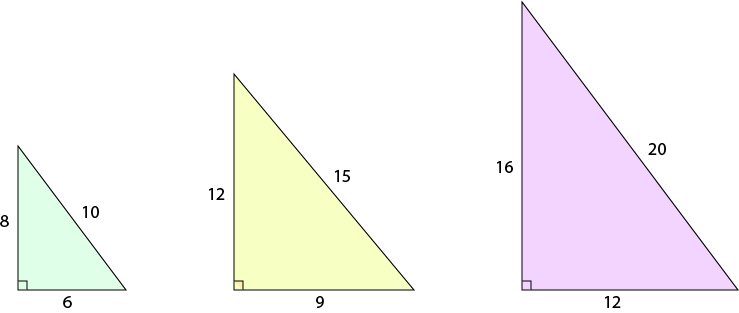### What About Sets of Sides that Aren't Multiples of 3,4, and 5?

Well, now things get more complicated, but the answer is still yes. First, we need to understand why 3,4, and 5 works.

## Pythagoras' Theorem

Pythagoras' Theorem states that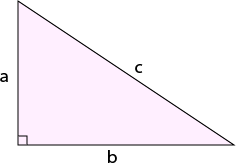In a right-angled triangle, the sum of the squares of the shorter sides ($a^2 + b^2$) is equal to the square of the longer side ($c^2$).

$a^2 + b^2 = c^2$

Pythagoras' theorem also works in reverse: if the sum of the squares of the shorter sides of a triangle is equal to the square of the longer side of the triangle, then the triangle is right angled.

Let's check out our 3-4-5 triangle: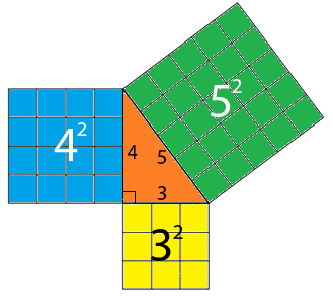For the 3-4-5 triangle,

$3^2 + 4^2 = 9 + 16 = 25 = 5^2$

So, yes, it works and our 3-4-5 triangle is right angled.

### So What About Those Other Side-Lengths

There are infinitely many of them! Any three positive numbers $a, b$ and $c$ that satisfy $a^2 + b^2 = c^2$ will give you a right-angled triangle. We call a set of three numbers satisfying this equation a Pythagorean Triad.

Here are a few more examples: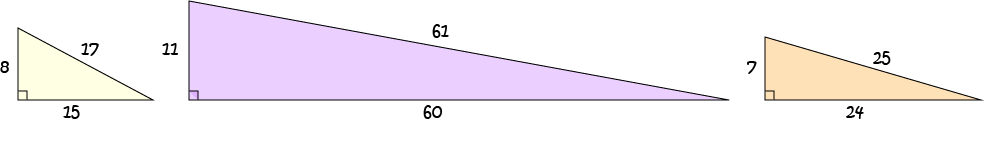Let's test them out:

• The 8-15-17 triangle:
\begin{align*} 8^2 + 15^2 &= 64 + 225\\ &= 289\\ &= 17^2 \end{align*}
So this one is right-angled.
• The 11-60-61 triangle:
\begin{align*} 11^2 + 60^2 &= 121 + 3,600\\ &= 2,721\\ &= 61 \end{align*}
So this one is right-angled too.
• Why not check the 7-24-5 triangle yourself?
There are many other Pythagorean triads. Why not see if you can find some more yourself that aren't multiples of 3, 4 and 5? If you want to learn more about Pythagorean triads, there's an article that will give you some further information.

### Description

In these chapters you will learn about plane geometry topics such as

• Area (Irregular polygons, plane shapes etc)
• Perimeter
• Conic sections (Circle, Ellipse, Hyperbola etc)
• Polygons (Congruent, polygons, similar, triangles etc)
• Transformations and symmetry (Reflection, symmetry, transformations etc)

etc

Even though these chapters are marked for Year 10 or higher students, several topics are for students in Year 8 or higher

### Audience

Year 10 or higher, suitable for Year 8 + students as well.

### Learning Objectives

You must be logged in as Student to ask a Question.